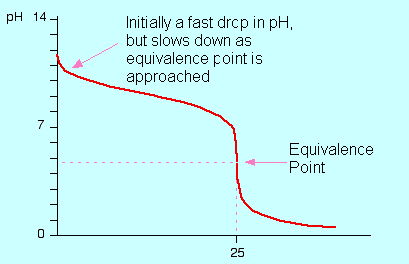## Strong Acid-Weak Base Titrations

#### Learning Objective

• Recall that strong acid-weak base titrations can be performed with either serving as the titrant.

#### Key Points

• The titration is typically performed as an acid into base.
• Following the titration with a pH meter in real time generates a curve showing the equivalence point.
• If the approximate pH of the equivalence point is known, a colorimetric indicator can be used in the titration.

#### Terms

• titrationThe determination of the concentration of some substance in a solution by slowly adding measured amounts of some other substance (normally using a burette) until a reaction is shown to be complete—for instance, by the color change of an indicator.
• bufferA solution used to stabilize the pH (acidity) of a liquid.
• equivalence pointThe point in a chemical reaction at which chemically equivalent quantities of acid and base have been mixed.

An example of a strong acid-weak base titration is the reaction between ammonia (a weak base) and hydrochloric acid (a strong acid) in the aqueous phase:

$NH_3 (aq) + HCl (aq) \rightarrow {NH_4^+}(aq) + Cl^-(aq)$

The acid is typically titrated into the base. A small amount of the acid solution of known concentration is placed in the burette (this solution is called the titrant). A known volume of base with unknown concentration is placed into an Erlenmeyer flask (the analyte), and, if pH measurements can be obtained via electrode, a graph of pH vs. volume of titrant can be made (titration curve).

In the case of titrating the acid into the base for a strong acid-weak base titration, the pH of the base will ordinarily start high and drop rapidly with the additions of acid. As the equivalence point is approached, the pH will change more gradually, until finally one drop will cause a rapid pH transition through the equivalence point. If a chemical indicator is used—methyl orange would be a good choice in this case—it changes from its basic to its acidic color.Titration of a weak base with a strong acidA depiction of the pH change during a titration of HCl solution into an ammonia solution. The curve depicts the change in pH (on the y-axis) vs. the volume of HCl added in mL (on the x-axis).

In strong acid-weak base titrations, the pH at the equivalence point is not 7 but below it. This is due to the production of a conjugate acid during the titration; it will react with water to produce hydronium (H3O+) ions.

In the example of the titration of HCl into ammonia solution, the conjugate acid formed (NH4+) reacts as follows:

$NH_4^+ + H_2O \rightarrow H_3O^+ + NH_3$